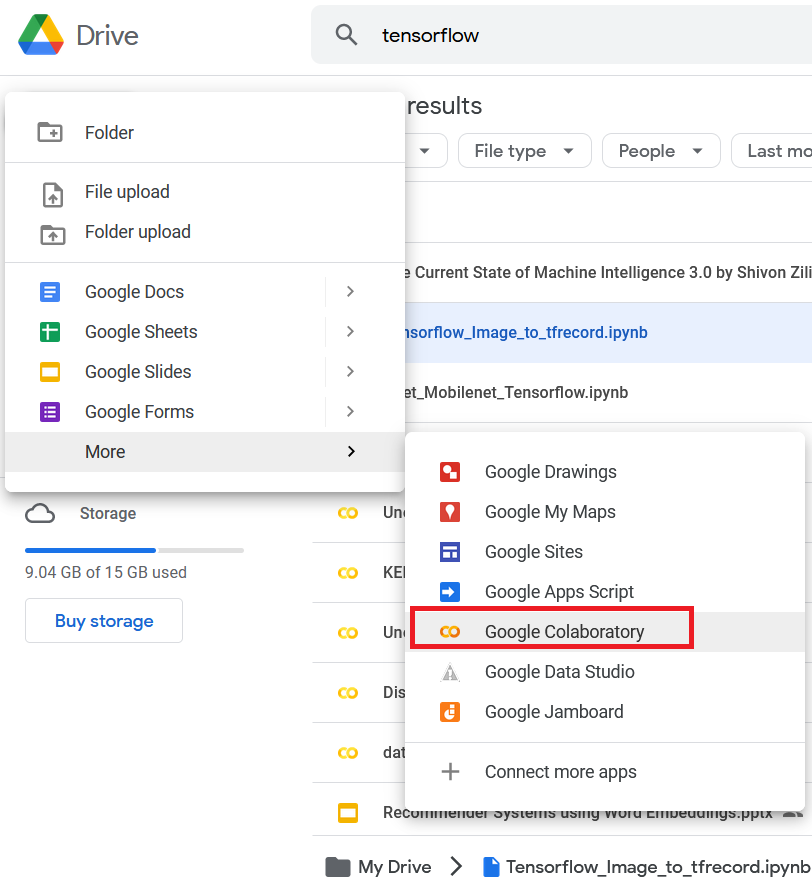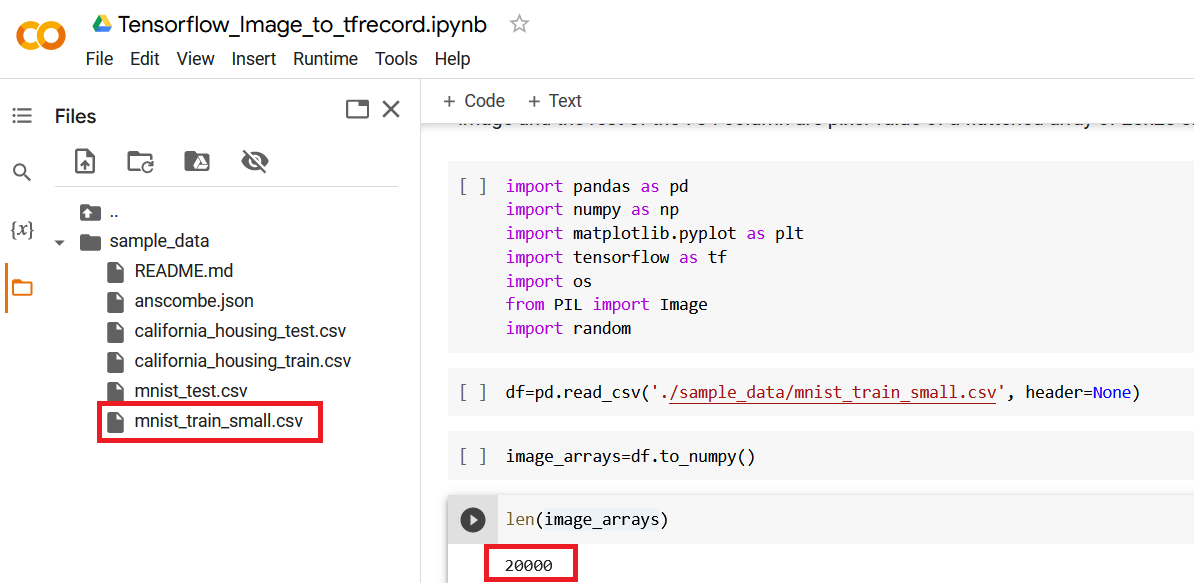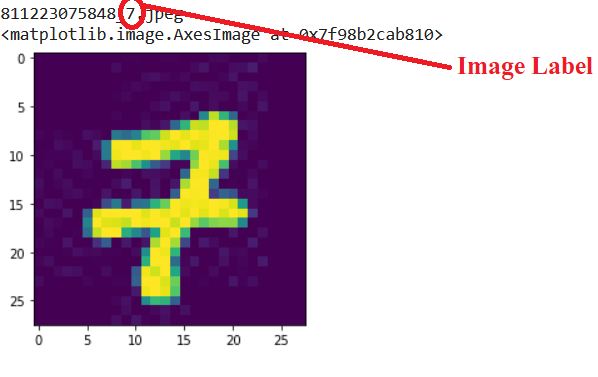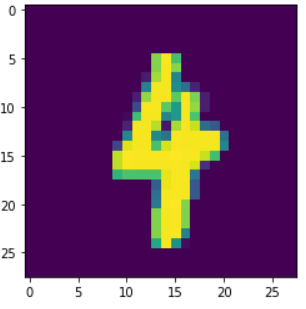# How to create tfrecord from image ndarray dataset

In this post we will create tfrecord files from images and the dataset that we will be using is google colab MNIST sample_data for training.

The raw dataset is a csv file,with 20,000 rows and 785 column. Each row represent an image with 784 columns that represents pixel value of a flattened 28x28 image array.

We will convert this csv dataset into two separate dataset, a numpyndarray and a Image dataset with 20000 images(jpeg).

Here are the steps that we need to follow for creating the tfrecord files:

Image Dataset:

• Create numpy ndarray from csv file
• Build JPEG images dataset for each row(image) in csv dataset
• Store each image as byte array to tfrecord file
• Parse tfrecord file and visualize the data stored

Let’s get started

## SetupGo to files tab and there you will find a default folder sample_data and mnist_train_small.csv file in it, this is our dataset file with 20,000 rows and 785 columns, the first column is the label and remaining 784 columns are pixel values of a 28x28 image## Create a Numpy ndarray from Image Pixels Dataframe

First we will read the csv file(mnist_train_small.csv) using pandas and load all the data in a pandas dataframe. After that convert the dataframe to a Numpy ndarray using to_numpy() function.

This function will yield a numpy.ndarray of size 20000x785, where the first column is the label of the image and the rest of the 784 column are pixel value of a flattened array of 28x28 size

``````import pandas as pd
import numpy as np
import matplotlib.pyplot as plt
import tensorflow as tf
import os
from PIL import Image
import random
``````
``````df=pd.read_csv('./sample_data/mnist_train_small.csv', header=None)
``````
``````image_arrays=df.to_numpy()
len(image_arrays)
``````
``````Out:
20000
``````

## Create Image dataset using image numpy ndarray

First, we need to store each image array as JPEG file with it’s label as file name. The filename is a random 12 digit number followed by label of the image.

``````for img in image_arrays[:100]:
filename = os.path.join('images', str(random.randint(100000000000,999999999999))+f'_{img}.jpeg')
Image.fromarray(np.uint8(np.reshape(img[1:], (28,28)))).save(filename)
``````

Lets load and visualize one of the image. This ensures the images are stored successfully

``````images=os.listdir('images')
n=62
print(images[n])
plt.imshow(im)
``````## Create function for dictionary mapping the feature name to the tf.train.Example-compatible data type

Fundamentally, a tf.train.Example is a {“string”: tf.train.Feature} mapping.

The tf.train.Feature message type can accept one of the following three types:

• tf.train.BytesList
• tf.train.FloatList
• tf.train.Int64List

The following functions can be used to convert a value to a type compatible with tf.train.Example.

Note that each function takes a scalar input value and returns a tf.train.Feature containing one of the three list types above.

If the input type for a function does not match one of the coercible types stated above, the function will raise an exception

Source: Tensorflow official documentation

``````def image_feature(value):
"""Returns a bytes_list from a string / byte."""
return tf.train.Feature(
bytes_list=tf.train.BytesList(value=[tf.io.encode_jpeg(value).numpy()])
)

def bytes_feature(value):
"""Returns a bytes_list from a string / byte."""
return tf.train.Feature(bytes_list=tf.train.BytesList(value=[value]))

def float_feature(value):
"""Returns a float_list from a float / double."""
return tf.train.Feature(float_list=tf.train.FloatList(value=[value]))

def int64_feature(value):
"""Returns an int64_list from a bool / enum / int / uint."""
return tf.train.Feature(int64_list=tf.train.Int64List(value=[value]))

def float_feature_list(value):
"""Returns a list of float_list from a float / double."""
return tf.train.Feature(float_list=tf.train.FloatList(value=value))
``````

In this section, we will create a feature map for the values that we want to store in the tfrecord file.

We would like to store the following data for each image Image byte array label

Each value needs to be converted to tf.train.Feature containing one of the 3 compatible types, using one of the functions below.

The create_example function is built to create a dictionary mapping the feature name to the tf.train.Example-compatible data type and to create a Features message using tf.train.Example.

``````def create_example(image, label):
feature = {
"image": bytes_feature(image),
"label": int64_feature(label)
}
return tf.train.Example(features=tf.train.Features(feature=feature))
``````

## Create tfrecord file using TFRecordWriter

Since we have 20K images, we will write only 3000 image data in one file and eventually there will be 7 tfrecord files created and the last file will have 2K records.

The num_samples is to define how many image samples we need in each file and num_tfrecords gives the number of tfrecord files to be created

``````tfrecords_dir = "./tfrecord"
num_samples = 3000
num_images = len(image_arrays)
num_tfrecods = num_images // num_samples
if num_images % num_samples:
num_tfrecods += 1  # add one record if there are any remaining samples

if not os.path.exists(tfrecords_dir):
os.makedirs(tfrecords_dir)
``````

This is a small code snippet that converts the image ndarray to bytes and calls the create_example() function to create a dictionary with feature message and serialize the data and finally write them to tfrecord file using TFRecordWriter

``````for tfrec_num in range(num_tfrecods):
samples = image_arrays[(tfrec_num * num_samples) : ((tfrec_num + 1) * num_samples)]
with tf.io.TFRecordWriter(
tfrecords_dir + "/file_%.2i-%i.tfrec" % (tfrec_num, len(samples))
) as writer:
for sample in samples:
# image=np.reshape(image,(28,28))
img = sample[1:].tobytes()
label = sample
example = create_example(img, label)
writer.write(example.SerializeToString())
``````

You can see here there are 7 files created and each file contains 3K image samples and its label

``````!ls tfrecord
``````
``````Out:
file_00-3000.tfrec  file_02-3000.tfrec	file_04-3000.tfrec  file_06-2000.tfrec
file_01-3000.tfrec  file_03-3000.tfrec	file_05-3000.tfrec
``````

## Parse tfrecord file and visualize stored images

Now let’s parse this tfrecord file and load into tf.data.TFRecordDataset and verify if the images and it’s label are stored correctly or not

To parse the input tf.train.Example proto we need to create a dictionary describing the features and a _parse_image_function()

``````image_feature_description = {
'image': tf.io.FixedLenFeature([], tf.string),
'label': tf.io.FixedLenFeature([], tf.int64)
}

def _parse_image_function(example_proto):
return tf.io.parse_single_example(example_proto, image_feature_description)

train_dataset = train_data.map(_parse_image_function)
``````

The tf.data.TFRecordDataset creates dataset comprising records from the tfrecord files.

This dataset loads TFRecords from the files as bytes and Parsing and decoding can be done by applying dataset.map.

We will map _parse_image_function() to parse the records

``````import numpy as np
import IPython.display as display

raw_dataset = tf.data.TFRecordDataset(f"{tfrecords_dir}/file_01-{num_samples}.tfrec")
parsed_dataset = raw_dataset.map(parse_tfrecord_fn)

for image_features in parsed_dataset.take(2):
print(image_features['label'].numpy())
image_raw = np.frombuffer(image_features['image'].numpy())
im=np.reshape(image_raw,(28,28))
plt.imshow(im)
``````Tags:

Categories:

Updated: